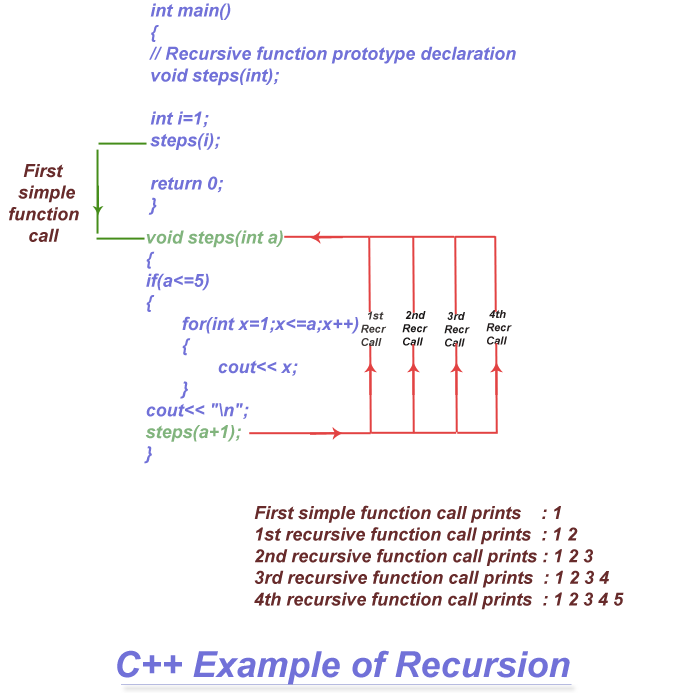< Prev
Next >

# C++ Recursion

Recursion is a technique when a function calls itself from its own code. Using C++ language, we can execute this technique by which a function will call itself to do a specific task. The function that can call itself from its own code is said to be a "recursive function".

To understand the technique of recursion, we will create two programs to do the same task of printing a numbers from 1 to 5 in successive steps. For example -
• Printing 1 in the first line.
• Printing 1 and 2 in the second line.
• Printing 1, 2 and 3 in the third line.
• Printing 1, 2, 3 and 4 in the fourth line
• Printing 1, 2, 3, 4 and 5 in the fifth line.

In the first program, we will see a simple function(a non-recursive function) doing the task and in the second program we will implement a recursive function doing the same task.

## A simple function(non-recursive) to print numbers from 1 to 5 in steps

Let's see the first program which implements the a simple non-recursive function , which has used two for loops(one nested in another) to implement the task of printing the numbers from 1 to 5 in steps, as mentioned above.

``````// A simple(non-recursive) function

#include<iostream>

using namespace std;

int main()
{
// function prototype declaration
void steps(int);

int i=1;
steps(i);

return 0;
}

//function defintion
void steps(int a)
{

for(int x=a;x<=5;x++)
{
for(int y=1;y<=x;y++)
{
cout<< y;
}
cout<< "\n";
}
}``````

## Output

``````1
1 2
1 2 3
1 2 3 4
1 2 3 4 5``````

## Recursive function to print numbers from 1 to 5 in steps

Let's see the second program that creates a recursive function with the namesteps, which calls itself to print the numbers from 1 to 5 in successive steps.

``````// A simple recursive function

#include<iostream>

using namespace std;

int main()
{

// Recursive function prototype declaration
void steps(int);

int i=1;
steps(i);

return 0;
}

//Recursive function
void steps(int a)
{
if(a<=5)
{
for(int x=1;x<=a;x++)
{
cout<< x;
}
cout<< "\n";

steps(a+1);		//Recursive function calling itself from its own code
}

}``````

## Output

``````1
1 2
1 2 3
1 2 3 4
1 2 3 4 5``````

## Program Analysis

Let us understand our recursion program with a diagram -As you can see in the self-explanatory diagram, the function steps() is called in successions, like -
• Starting the program, the main() function calls function steps(), which prints 1.
• Next time, the steps() function calls itself recursively for the first time, which prints 1,2
• Next time, the steps() function calls itself recursively for the second time, which prints 1,2,3
• Next time, the steps() function calls itself recursively for the third time, which prints 1,2,3,4
• Finally, the steps() function calls itself recursively for the fourth time, which prints 1,2,3,4,5

## Another example of Recursion

In the upcoming code example, we are going to find the factorial of 5 using a recursive function that calls itself.

``````// Recursion Example - Finding Factorial

#include<iostream>

using namespace std;

int main()
{

// function prototype declaration
int factor(int);

int num = 5;
int result = factor(5);

cout<< "Factorial of 5 is " << result;

return 0;
}

int factor(int a)
{
int result;

if(a==1)
return 1;
else
result = a * factor(a-1); // recursive function factor has called itself

return result;
}``````

## Output

``Factorial of 5 is 120``